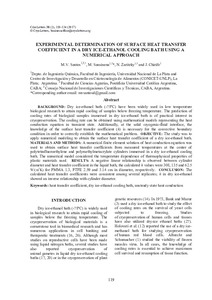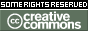Please use this identifier to cite or link to this item: `https://repositorio.uca.edu.ar/handle/123456789/15089`
 Título : Experimental determination of surface heat transfer coefficient in a dry ice-ethanol cooling bath using a numerical approach Autor : Santos, María V. Sansiñena, Marina Julia Zaritzky, Noemí Chirife, Jorge Palabras clave : TEMPERATURA; ENFRIAMIENTO; TRANSFERENCIA DE CALOR; ETANOL Fecha de publicación : 2017 Cita : Santos, M. V. et al. Experimental determination of surface heat transfer coefficient in a dry ice-ethanol cooling bath using a numerical approach [en línea]. Cryolettes. 2017, 38 (2). Disponible en: https://repositorio.uca.edu.ar/handle/123456789/15089 Resumen : Abstract: Background: Dry ice-ethanol bath (-78ºC) have been widely used in low temperature biological research to attain rapid cooling of samples below freezing temperature. The prediction of cooling rates of biological samples immersed in dry ice-ethanol bath is of practical interest in cryopreservation. The cooling rate can be obtained using mathematical models representing the heat conduction equation in transient state. Additionally, at the solid cryogenic-fluid interface, the knowledge of the surface heat transfer coefficient (h) is necessary for the convective boundary condition in order to correctly establish the mathematical problem. Objective: The study was to apply numerical modeling to obtain the surface heat transfer coefficient of a dry ice-ethanol bath. Materials and methods: A numerical finite element solution of heat conduction equation was used to obtain surface heat transfer coefficients from measured temperatures at the center of polytetrafluoroethylene and polymethylmetacrylate cylinders immersed in a dry ice-ethanol cooling bath. The numerical model considered the temperature dependence of thermophysical properties of plastic materials used. Results: A negative linear relationship is observed between cylinder diameter and heat transfer coefficient in the liquid bath, the calculated h values were 308, 135 and 62.5 W/(m2K) for PMMA 1.3, PTFE 2.59 and 3.14 cm in diameter, respectively. Conclusion: The calculated heat transfer coefficients were consistent among several replicates; h in dry ice-ethanol showed an inverse relationship with cylinder diameter. URI : https://repositorio.uca.edu.ar/handle/123456789/15089 ISSN : 0143-2044 Disciplina: INGENIERIA EN ALIMENTOS Derechos: Acceso abierto Appears in Collections: Artículos

###### Files in This Item:
File Description SizeFormat
experimental-determination-surface.pdf549,93 kBAdobe PDF#### Page view(s)

24
checked on Sep 26, 2023This item is licensed under a Creative Commons License